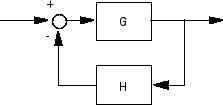Documentation

### This is machine translation

Mouseover text to see original. Click the button below to return to the English version of the page.

# inv

Invert models

## Syntax

```inv ```

## Description

`inv ` inverts the input/output relation

`$y=G\left(s\right)u$`

to produce the model with the transfer matrix $H\left(s\right)=G{\left(s\right)}^{-1}$.

`$u=H\left(s\right)y$`

This operation is defined only for square systems (same number of inputs and outputs) with an invertible feedthrough matrix D. `inv` handles both continuous- and discrete-time systems.

## Examples

Consider

`$H\left(s\right)=\left[\begin{array}{cc}1& \frac{1}{s+1}\\ 0& 1\end{array}\right]$`

At the MATLAB® prompt, type

```H = [1 tf(1,[1 1]);0 1] Hi = inv(H) ```

to invert it. These commands produce the following result.

```Transfer function from input 1 to output... #1: 1 #2: 0 Transfer function from input 2 to output... -1 #1: ----- s + 1 #2: 1 ```

You can verify that

```H * Hi ```

is the identity transfer function (static gain `I`).

## Limitations

Do not use `inv` to model feedback connections such asWhile it seems reasonable to evaluate the corresponding closed-loop transfer function ${\left(I+GH\right)}^{-1}G$ as

```inv(1+g*h) * g ```

this typically leads to nonminimal closed-loop models. For example,

```g = zpk([],1,1) h = tf([2 1],[1 0]) cloop = inv(1+g*h) * g ```

yields a third-order closed-loop model with an unstable pole-zero cancellation at `s` = `1`.

```cloop Zero/pole/gain: s (s-1) ------------------- (s-1) (s^2 + s + 1) ```

Use `feedback` to avoid such pitfalls.

```cloop = feedback(g,h) Zero/pole/gain: s ------------- (s^2 + s + 1) ```

﻿